• 内容简介频率域去噪带阻滤波器带通滤波器陷波滤波器最佳陷波滤波器 频率域去噪 为了节约读者时间，首先说明这一篇不会有任何代码只有个人的心得体会理由如下： 代码过于简单，像书上这样的例子这样的 自己并没有这类...
• 1. 频率域去噪基本实现思想： 首先将原始图像通过一些积分变换，将其变换到频率域，接着再通过频率域对其进行操作，得到的结果再反变换到空间域中，进而使图像得到增强。根据傅里叶频谱的特性可得到，图像的平均...
1. 频率域去噪基本实现思想：
首先将原始图像通过一些积分变换，将其变换到频率域，接着再通过频率域对其进行操作，得到的结果再反变换到空间域中，进而使图像得到增强。根据傅里叶频谱的特性可得到，图像的平均灰度级对应于频率为0成分，当从傅里叶变换的原点离开时，图像的慢变化分量对应着低频滤波，比如一幅图像中较平的区域；当再进一步离开原点时，较高的频率开始对应图像中变换越来越快的灰度级，它们反映了一幅图像中物体的边缘和灰度级突发改变和噪声部分的图像成分。频率域图像增强正是基于这种原理，通过对图像的傅里叶频谱进行低通滤波(使低频通过，使高频衰退)来过滤噪声，通过对图像的傅里叶频谱进行高通滤波(使高频通过，使低频衰退)使图像的边缘和轮廓更明显。
含噪图像信号进过小波变换后,噪声的小波系数比较大， 而图像的小波系数比较小，通过阈值，可以达到去噪的目的。   对于二维图像信号，由一维小波张成的二维小波并没有继承一维小波的优良特性，二维小波变换也不是最优的表示，就有了Curvelet,Contourlet,Wedgelet,Bandelet,Directionlet等多尺度的几何分析方法。

2. 从稀疏表示的角度看图像去噪：
含噪的图像信号$Y$包括两部分，干净图像信号$X$与噪声$\epsilon$。
$$Y=X+\epsilon$$
由于干净的图像信号是又一定的结构的，其结构特性与原子特性相吻合，故干净图像信号具有稀疏性（$\theta$稀疏）:
$$X=D\theta$$
而噪声是随机的，不相关，因此没有结构特性，$\epsilon$不能由字典$D$稀疏表示；
因此通过求解
$$\min \|Y-D\theta\|, \;\; s.t., \|\theta\|_0 <\mathrm{tol}$$
这样的原理可以达到图像去噪的目的。
稀疏表示又在稀疏领域开阔了一片空间，结合多尺度的基函数，可以构造出小波字典，混合字典，双冗余字典。用这些工具在去噪领域，取得了很好的性能。

3. 图像去噪与压缩感知
仔细分析信号去噪和压缩感知，会发现二者有相似之处（优化模型），但是二者出发点和落脚点都是不一样的，这导致二者的已知和未知的信息都是不尽一致的；
其实压缩感知本来就不是用于图像恢复的，而是用于信号的采样，它的目的是克服香农均匀采用定理的高采样频率问题提出的，对于亚采样也是可以精确重构原始信号，换句话说：压缩感知是用来采样原始信息，然后降低存储。至于用压缩感知去恢复缺失图像的信息（或者去噪），它可以做，只不过目的性略显牵强。

转载于:https://www.cnblogs.com/mathlife/p/9092545.html
展开全文• 我们知道图像的经典去噪方法主要有两大类：一类是基于空间域的处理方法，一类是基于频域的处理方法...因此图像变换域去噪算法的基本思想其实就是首先进行某种变换，将图像从空间域转换到变换域，然后从频率上把噪声...
我们知道图像的经典去噪方法主要有两大类：一类是基于空间域的处理方法，一类是基于频域的处理方法。前面三节讲到的欧式基于空间域的处理方法，基于频域的处理方法主要是用滤波器，把有用的信号和干扰信号分开，它在有用信号和干扰信号的频谱没有重叠的前提下，才能把有用信号和干扰信号完全分开，但实际上很难分开。
因此图像变换域去噪算法的基本思想其实就是首先进行某种变换，将图像从空间域转换到变换域，然后从频率上把噪声分为高中低频噪声，用这种变换域的方法就可以把不同频率的噪声分离，之后进行反变换将图像从变换域转换到原始空间域，最终达到去除图像噪声的目的。
（一）傅里叶变换
傅立叶变换用于分析各种滤波器的频率特性，
对于一幅图像来说在分析其频率特性时，它的边缘，突出部分以及颗粒噪声往往代表图像信号的高频分量，而大面积的图像背景区则代表图像信号的低频分量。因此，使用滤波的方法滤除其高频部分也就可以去除噪声，使得图像得到一定的平滑。
图像是二维离散的，连续与离散都可以用傅里叶进行变换，那么二维信号无非就是在x方向与y方向都进行一次一维的傅里叶变换得到。所有图像的频率构成都认为是这样的，那么不同的就是一幅图的振幅与相位了，也就是说在opencv或者matlab下对图像进行傅里叶变换后其实是可以得到图像的振幅图与相位图的，而想把图像从频域空间恢复到时域空间，必须要同时有图像的振幅图与相位图才可以，缺少一个就恢复的不完整。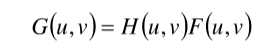其中，F(u,v)是含噪声图像的傅里叶变换，G(u,v)是平滑后图像的傅里叶变换，H(u,v)是低通滤波器传递函数。处理过程如下图所示：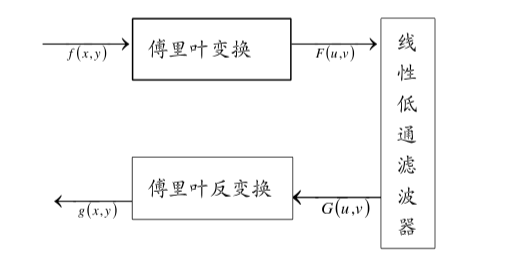利用numoy包进行傅里叶变换
np.fft.fft2()
第一个参数是输入图像，它是灰度图像
第二个参数是可选的，它决定了输出数组的大小,如果它大于输入图像的大小，则输入图像在计算FFT之前填充了0.如果它小于输入图像，输入图像将被裁剪,如果没有参数传递，输出数组的大小将与输入相同.
一旦得到结果，零频率分量（DC分量）将位于左上角。 如果要将其置于中心位置，则需要在两个方向上将结果移动N2.np.fft.fftshift(),一旦你找到频率变换，你就能找到大小谱.
import cv2
import numpy as np
from matplotlib import pyplot as plt

f=np.fft.fft2(img)
fshift=np.fft.fftshift(f)
magnitude_spectrum=20*np.log(np.abs(fshift))  #取绝对值是为了将复数变换成实数，
#取对数的目的是将数据变化到较小的范围（比如0--255）

plt.subplot(121),plt.imshow(img, cmap = 'gray')
plt.title('Input Image'), plt.xticks([]), plt.yticks([])
plt.subplot(122),plt.imshow(magnitude_spectrum, cmap = 'gray')
plt.title('Magnitude Spectrum'), plt.xticks([]), plt.yticks([])
plt.show()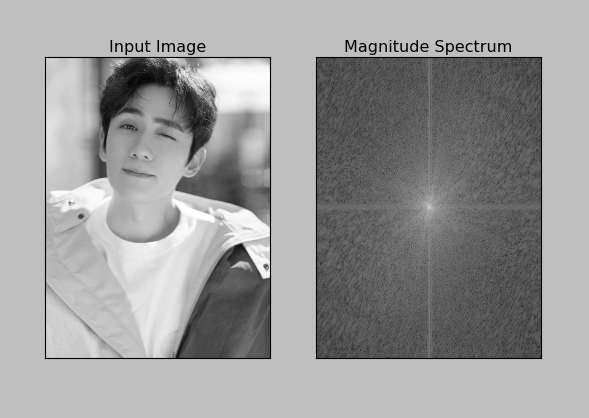但是上图并没有什么含义，显示出来的可以看成是频域中图像的振幅信息，但是没有相位信息，图像的相位ϕ=atan(实部/虚部），numpy包中自带一个angle函数可以直接根据复数的实部和虚部求出角度（默认是弧度）。
import cv2
import numpy as np
from matplotlib import pyplot as plt

f=np.fft.fft2(img)
fshift=np.fft.fftshift(f)

ph_f=np.angle(f)
ph_fshift=np.angle(fshift)

plt.subplot(121),plt.imshow(ph_f, cmap = 'gray')
plt.title('original'), plt.xticks([]), plt.yticks([])
plt.subplot(122),plt.imshow(ph_fshift, cmap = 'gray')
plt.title('center'), plt.xticks([]), plt.yticks([])
plt.show()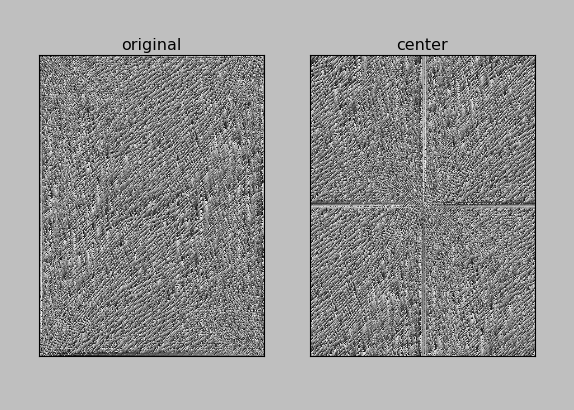上图就是图像上每个像素点对应的相位图，理解就是偏移的角度。
下面介绍一下频域下的低通滤波器，高通滤波器，带通带阻滤波器。
1.高通滤波器
图像在变换加移动中心后，从中间到外面，频率上依次是从低频到高频，因此我们如果把中间一小部分去掉，就相当于高通滤波器：
黑色为0，白色为1，把这个模板和图像的傅里叶变换的频域矩阵相乘就得到了高通滤波。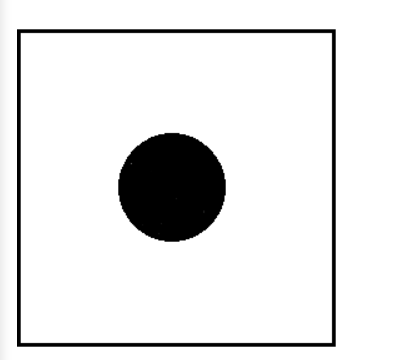import cv2
import numpy as np
from matplotlib import pyplot as plt
f=np.fft.fft2(img)
fshift=np.fft.fftshift(f)
magnitude_spectrum=20*np.log(np.abs(fshift))  #取绝对值是为了将复数变换成实数，
#取对数的目的是将数据变化到较小的范围（比如0--255）
rows,cols=img.shape
crow,ccol=int(rows/2),int(cols/2)

f_ishift=np.fft.ifftshift(fshift)
img_back=np.fft.ifft2(f_ishift) # 傅里叶逆变换
img_back=np.abs(img_back) # 得到的是复数会无法显示

plt.subplot(221),plt.imshow(img, cmap = 'gray')
plt.title('original'), plt.xticks([]), plt.yticks([])
plt.subplot(222),plt.imshow(img_back, cmap = 'gray')
plt.title('Image after HPF'), plt.xticks([]), plt.yticks([])
plt.show()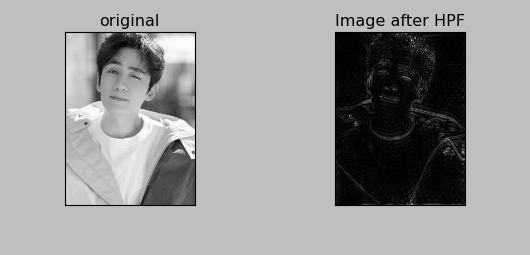可以看出，高通滤波器有利于提取图像的轮廓。这是因为图像的轮廓或者边缘或者噪声处，灰度变化强烈，那么在它们经过傅里叶变换后，就会变成高频信号。
2.低通滤波器
低通滤波器就是把上述模板的1变成0，0变成1：
import cv2
import numpy as np
from matplotlib import pyplot as plt

f=np.fft.fft2(img)
fshift=np.fft.fftshift(f)
magnitude_spectrum=20*np.log(np.abs(fshift))  #取绝对值是为了将复数变换成实数，
#取对数的目的是将数据变化到较小的范围（比如0--255）

rows,cols=img.shape
crow,ccol=int(rows/2),int(cols/2)

f_ishift=np.fft.ifftshift(fshift)
img_back=np.fft.ifft2(f_ishift) # 傅里叶逆变换
img_back=np.abs(img_back) # 得到的是复数会无法显示

plt.subplot(221),plt.imshow(img, cmap = 'gray')
plt.title('original'), plt.xticks([]), plt.yticks([])
plt.subplot(222),plt.imshow(img_back, cmap = 'gray')
plt.title('Image after LPF'), plt.xticks([]), plt.yticks([])
plt.show()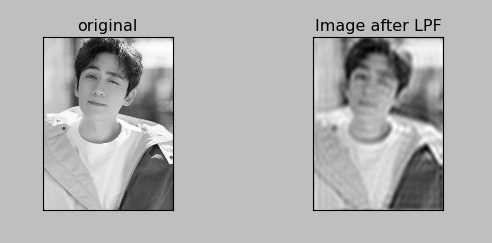可以看到低通滤波后除了轮廓模糊外，基本没变化，这是因为图像的主要信息都集中到了低频上。
3.带通滤波器
import cv2
import numpy as np
from matplotlib import pyplot as plt

f=np.fft.fft2(img)
fshift=np.fft.fftshift(f)

rows,cols=img.shape
crow,ccol=int(rows/2),int(cols/2)

f_ishift=np.fft.ifftshift(fshift)
img_back=np.fft.ifft2(f_ishift) # 傅里叶逆变换
img_back=np.abs(img_back) # 得到的是复数会无法显示

plt.subplot(221),plt.imshow(img, cmap = 'gray')
plt.title('original'), plt.xticks([]), plt.yticks([])
plt.subplot(222),plt.imshow(img_back, cmap = 'gray')
plt.title('Image after BPF'), plt.xticks([]), plt.yticks([])
plt.show()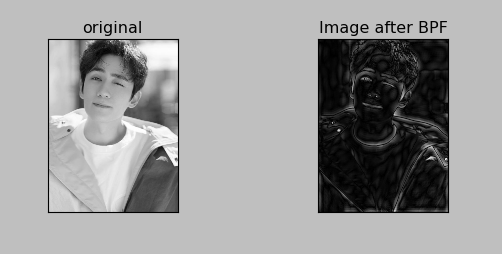带通滤波的效果，既保留了一部分低频，也保留了一部分高频。
（二）基于小波变换的图像去噪技术
傅里叶变换对于非平稳过程有局限性：它只能获取一段信号总体上包含哪些频率的成分，但是对各个成分出现的时刻不清楚。因此时域相差很大的两个信号，可能频谱图一样。
但是对于一个非平稳信号，只知道包含哪些频率成分时不够的，我们还想知道各个成分出现的时间，知道信号频率随时间变化的情况，各个时刻的顺时频率及其幅值----时频分析。
小波把傅里叶变换的基换了，将无限长的三角函数基换成了有限长的会衰减的小波基。这样不仅能够获取频率，还可以定位到时间了。
1.小波阈值去噪介绍
主要思想是经过小波变换后图像和噪声的统计特性不同，其中图像本身的小波系数具有较大幅值，主要集中在高频，噪声小波系数幅值较小，并且存在于小波变换后的所有系数中。因此设置一个阈值门限来进行分离。
通常在去噪时，不处理含有大量图像能量的低通系数，只是就单个高通部分进行处理。因此不能只进行一次阈值去噪，还需要对低频部分进行阈值去噪和小波分解，直到估计噪声和实际图像的偏差值最小。一般进行3-4层小波分解和去噪就可以了。
2.小波阈值去噪方法
算法的基本过程为：
①对原始信号进行小波分解
②对变换后的小波系数进行阈值处理，得到估计小波系数
③根据估计小波系数进行小波重构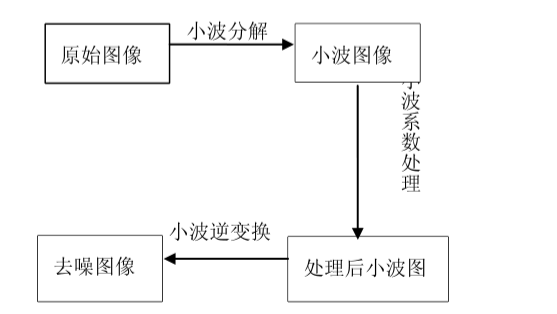3.小波阈值去噪基本问题

小波基的选择：通常我们希望所选取的小波满足以下条件：正交性、高消失矩、紧支性、对称性或反对称性。但事实上具有上述性质的小波是不可能存在的，因为小波是对称或反对称的只有Haar小波，并且高消失矩与紧支性是一对矛盾，所以在应用的时候一般选取具有紧支的小波以及根据信号的特征来选取较为合适的小波。
阈值的选择：直接影响去噪效果的一个重要因素就是阀值的选取，不同的阈值选取将有不同的去噪效果。目前主要有通用阈值（VisuShrink）、SureShrink阈值、Minimax阈值、BayesShrink阈值等。
阈值函数的选择：阈值函数是修正小波系数的规则，不同的反之函数体现了不同的处理小波系数的策略。最常用的阈值函数有两种：一种是硬阈值函数，另一种是软阈值函数。还有一种介于软、硬阈值函数之间的Garrote函数。

利用阈值函数pywt.threshold: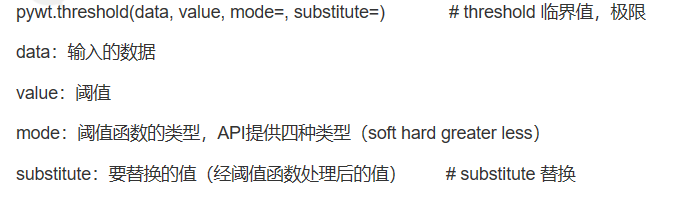import pywt
import numpy as np
import pandas as pd
import matplotlib.pyplot as plt
import math

# np.linspace()函数用于在指定的间隔内返回均匀间隔的数字。
data=np.linspace(1,10,10)
#[1. 2. 3. 4. 5. 6. 7. 8. 9. 10.]

# pywt.threshold(data,value,mode,substitute) mode 模式有四种，soft,hard,greater,less;substitute是替换值

data_soft=pywt.threshold(data=data,value=6,mode='soft',substitute=12)
# soft模式把小于6的值设置为12，大于等于6的值全部减6
#[12. 12. 12. 12. 12. 0. 1. 2. 3. 4.]

data_hard=pywt.threshold(data=data,value=6,mode='hard',substitute=12)
# hard模式把小于6的值设置为12，其余的值不变
#[12. 12. 12. 12. 12. 6. 7. 8. 9. 10.]

data_greater=pywt.threshold(data,6,'greater',12)
# 把小于6的值设为12，大于等于阈值的值不变
#[12. 12. 12. 12. 12. 6. 7. 8. 9. 10.]

data_less=pywt.threshold(data,6,'less',12)
# 把大于6的值设为12，小于等于阈值的值不变
#[1. 2. 3. 4. 5. 6. 12. 12. 12. 12. 12. ]

4.小波去噪实验
pywt.dwt_max_level(data_len,filter_len):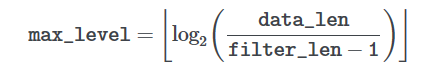pywt.wavedec(data, wavelet, mode=‘symmetric’, level=None, axis=-1)
data:输入数据，wavelet：使用的小波，level：分解级别，默认为dwt_max_len
返回：[cA_n, cD_n, cD_n-1, …, cD2, cD1]
n代表分解的级别，第一个cA_n代表的是近似分解系数，接下来的元素cD_n-cD1代表的是系数数组的详细信息
import pywt
import numpy as np
import pandas as pd
import matplotlib.pyplot as plt
import math

data=np.linspace(1,10,10)

# Get Data
ecg=pywt.data.ecg() # 生成心电信号
index=[]
data=[]
for i in range(len(ecg)-1):
X=float(i)
Y=float(ecg[i])
index.append(X)
data.append(Y)

# Create wavelet object and define parameters
w=pywt.Wavelet('db8') # 创建一个小波对象 选用Daubechies8小波
#  Family name:    Daubechies
#  Short name:     db
#  Filters length: 16  #滤波器长度为16
#  Orthogonal:     True  #正交
#  Biorthogonal:   True  #双正交
#  Symmetry:       asymmetric  # 对称性：不对称
#  DWT:            True  #离散小波变换
#  CWT:            False
maxlev=pywt.dwt_max_level(len(data),w.dec_len)  # 计算最大有用的分解级别（data_len,filter_len)
print("maximum level is " + str(maxlev))
threshold=0.04 # Threshold for filtering

# Decompose into wavelet components,to the level selected
coeffs=pywt.wavedec(data,'db8',level=maxlev)

plt.figure()
for i in range(1,len(coeffs)):
coeffs[i]=pywt.threshold(coeffs[i],threshold*max(coeffs[i]))  # 将噪声滤波

datarec=pywt.waverec(coeffs,'db8')  # 将信号进行小波重构

mintime=0
maxtime=mintime+len(data)+1

plt.figure()
plt.subplot(2,1,1)
plt.plot(index[mintime:maxtime],data[mintime:maxtime])
plt.xlabel('time(s)')
plt.ylabel('microvolts (uV)')
plt.title("Raw signal")
plt.subplot(2,1,2)
plt.plot(index[mintime:maxtime],datarec[mintime:maxtime-1])
plt.xlabel('time(s)')
plt.ylabel('microvolts (uV)')
plt.title("De-noised signal using wavelet techniques")

plt.tight_layout()
plt.show()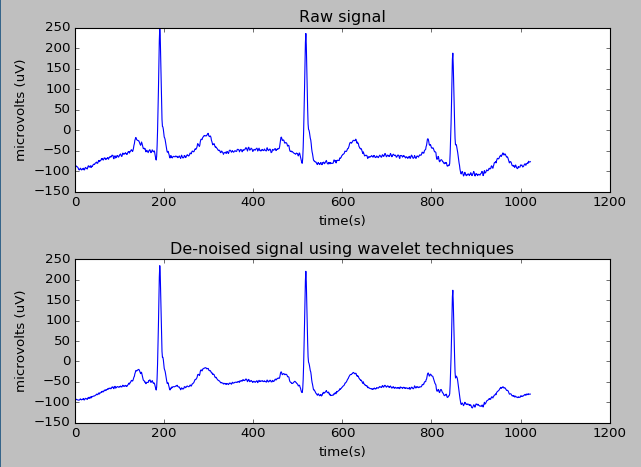参考链接：
Python小波变换去噪
图像滤波和傅里叶变换


展开全文• 翻阅冈萨雷斯《数字图像处理》这本教材和上课老师讲的内容，发现陷波滤波器可以用来在频率域去除指定噪声，是一种很有用的选择性滤波器，很适用于这张图片。陷波滤波器可以用中心已被平移到陷波滤波器中心的高通...
图片分析

第一眼看到这张图片，发现这张图片的噪声是有规则的，都是一条一条的。翻阅冈萨雷斯《数字图像处理》这本教材和上课老师讲的内容，发现陷波滤波器可以用来在频率域去除指定噪声，是一种很有用的选择性滤波器，很适用于这张图片。陷波滤波器可以用中心已被平移到陷波滤波器中心的高通滤波器的乘积来构造。因此，本次作业我用了两种高通滤波器来构造陷波滤波器，一种是布特沃斯高通滤波器，另外一种是高斯高通滤波器，最后分析比较了这两种滤波器的效果，并进行了一个总结。

高斯高通滤波器代码(matlab)

function [] = t2(I)
%   高斯高通陷波滤波器
figure,imshow(I);
title('原图像');
s=fftshift(fft2(I));
[M,N]=size(s);

figure,imshow(log(abs(s)),[]);
title('原图所得频谱');

gap=2;  %两个相邻的圆的间距，可根据需要进行调整
Do=15;  %可根据需要自行调整
row=round(M/2);

left=row-15;
right=row+15;

first=0;  %对变量进行初始化
next=0;
circleX=0;
circleY=0;

for i=1:M
for j=1:N
if i<=left || i>=right
if j>240 && j<270         %这部分区域对应噪声的区域，可根据需要进行微小调整

%下面这段代码的作用主要是找各个小圆的圆心
if i>(circleX+gap)
first=1;
next=1;
end
if first==0
circleX=gap;
circleY=256;
else
if next==1
next=0;  %初始化
circleX=circleX+2*gap;
circleY=256;
end
end

total=sqrt((i-circleX)^2+(j-circleY)^2);  %计算到小圆中心点的距离
s(i,j)=s(i,j)*(1-exp((-total*total)/(2*Do*Do))); % 高斯高通陷波滤波器
end
end
end
end

figure,imshow(log(abs(s)),[]);
title('高斯高通陷波滤波器处理后所得频谱');
s=uint8(real(ifft2(ifftshift(s))));
figure,imshow(s);
title('高斯高通陷波滤波器处理后的图');

end

布特沃斯高通滤波器

function [] = t1(I)
%T1 2阶布特沃斯陷波滤波器
figure,imshow(I);
title('原图像');
s=fftshift(fft2(I));
[M,N]=size(s);

figure,imshow(log(abs(s)),[]);
title('原图所得频谱');

gap=2;  %两个相邻的圆的间距，可根据需要进行调整
Do=15;  %截止频率
row=round(M/2);

left=row-15;
right=row+15;

first=0;  %初始化
next=0;
circleX=0;
circleY=0;

for i=1:M
for j=1:N
if i<=left || i>=right
if j>240 && j<270         %这部分区域对应噪声的区域，可根据需要进行微小调整

%下面这段代码的作用主要是找各个小圆的圆心
if i>(circleX+gap)
first=1;
next=1;
end
if first==0
circleX=gap;
circleY=256;
else
if next==1
next=0;  %初始化
circleX=circleX+2*gap;
circleY=256;
end
end

total=sqrt((i-circleX)^2+(j-circleY)^2);   %计算到小圆中心点的距离
s(i,j)=s(i,j)*1/(1+(Do/total)^4);  %截止频率为Do的2阶布特沃斯陷波滤波器
end
end
end
end

figure,imshow(log(abs(s)),[]);
title('布特沃斯陷波滤波器处理后所得频谱');
s=uint8(real(ifft2(ifftshift(s))));
figure,imshow(s);
title('布特沃斯陷波滤波器处理后的图');
end
总结

不管是2阶布特沃斯陷波滤波器还是高斯高通陷波滤波器，对图片的处理效果都不错。比较来说，通过对频谱图的分析，高斯高通陷波滤波器在去除噪声的同时，对图片的处理更平滑一些，效果更好一些。
通过这次作业，最大的收获在于对傅里叶变换的理解。傅里叶变换是从时域到频率域的转换，很多情况下，在时域处理内容比较复杂，而在频率域就变得很简单，处理之后再通过反傅里叶变换转换回来。
其次，我明白了一个道理。数字图像处理中，滤波器的种类有很多，每种滤波器都有自己的特点，或者说，每种特定的滤波器只适用于处理特定的内容。哪怕是同一种滤波器，对不同的图片处理效果都不一样，参数的修改很重要，对于怎么修改参数，取决于具体的应用和自身需要。我慢慢的爱上了这门课，非常有意思。


展开全文• 最近通过研究了一下频率域滤波，并试着用OpenCV实现，但是OpenCV并没有像MATLAB中 fftshift 这样的中心化操作，所以我写了一个完成频率域滤波的函数，以后用频率域滤波的时候在主函数中调用即可。当然，水平有限，...
  最近由于作业原因，试着用OpenCV实现频率域滤波，但是OpenCV中并没有像MATLAB中fftshift这样的中心化操作，所以我写了一个频率域滤波的函数，以后用频率域滤波的时候在主函数中调用即可。当然，水平有限，编写的代码并不优美，有问题请大家留言批评指正。  在这里我不介绍傅里叶变换，频率域滤波和高斯低通滤波器的原理，想必大家已经有了大概了解，本文关注于OpenCV中的代码实现。 废话少说，先上一张例图：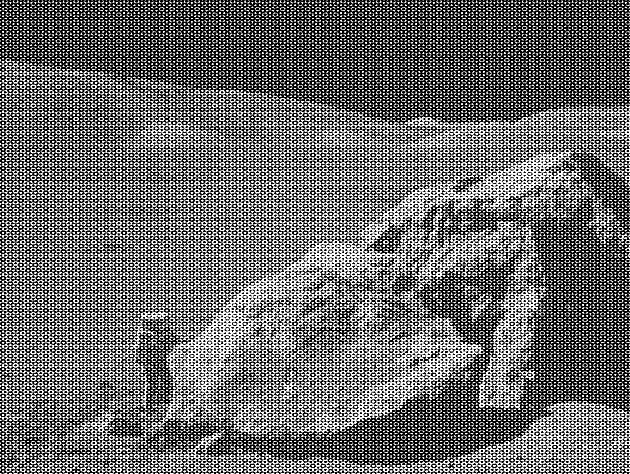这幅图像的噪声为周期性噪声，带用阻滤波器或者陷波滤波器去噪较好，这里用高斯低通去噪，效果一般，周期性没有完全去除。下面是频率域滤波的流程1、计算原图像的DFT，得到F（U，V）;2、将频谱F（U，V）的零频点移到中心位置;3、计算滤波器函数H（U，V）与上步结果F（U，V）的乘积G（U，V）;4、将上一步结果G（U，V）的零频点移回;（在OpenCV中没有这步也没关系，原因待解）5、计算上步的IDFT，得到g（X，Y）;6、取g（X，Y）的模作为结果。下面根据上述步骤编写代码如下：//**************************************
//频率域滤波——以高斯低通为例
//**************************************
#include<opencv2/opencv.hpp>
#include<iostream>

using namespace std;
using namespace cv;
Mat gaussianlbrf(Mat scr,float sigma);//高斯低通滤波器函数
Mat freqfilt(Mat scr,Mat blur);//频率域滤波函数

int main()
{
imshow("原图",input);
int w=getOptimalDFTSize(input.cols);  //获取进行dtf的最优尺寸
int h=getOptimalDFTSize(input.rows); //获取进行dtf的最优尺寸

imshow("结果图 sigma=40",out);

waitKey(0);
return 0;
}

//*****************高斯低通滤波器***********************
Mat gaussianlbrf(Mat scr,float sigma)
{
Mat gaussianBlur(scr.size(),CV_32FC1); //，CV_32FC1
float d0=2*sigma*sigma;//高斯函数参数，越小，频率高斯滤波器越窄，滤除高频成分越多，图像就越平滑
for(int i=0;i<scr.rows ; i++ )
{
for(int j=0; j<scr.cols ; j++ )
{
float d=pow(float(i-scr.rows/2),2)+pow(float(j-scr.cols/2),2);//分子,计算pow必须为float型
gaussianBlur.at<float>(i,j)=expf(-d/d0);//expf为以e为底求幂（必须为float型）
}
}
imshow("高斯低通滤波器",gaussianBlur);
return gaussianBlur;
}

//*****************频率域滤波*******************
Mat freqfilt(Mat scr,Mat blur)
{
//***********************DFT*******************
Mat plane[]={scr, Mat::zeros(scr.size() , CV_32FC1)}; //创建通道，存储dft后的实部与虚部（CV_32F，必须为单通道数）
Mat complexIm;
merge(plane,2,complexIm);//合并通道 （把两个矩阵合并为一个2通道的Mat类容器）
dft(complexIm,complexIm);//进行傅立叶变换，结果保存在自身

//***************中心化********************
split(complexIm,plane);//分离通道（数组分离）

int cx=plane.cols/2;int cy=plane.rows/2;//以下的操作是移动图像  (零频移到中心)
Mat part1_r(plane,Rect(0,0,cx,cy));  //元素坐标表示为(cx,cy)
Mat part2_r(plane,Rect(cx,0,cx,cy));
Mat part3_r(plane,Rect(0,cy,cx,cy));
Mat part4_r(plane,Rect(cx,cy,cx,cy));

Mat temp;
part1_r.copyTo(temp);  //左上与右下交换位置(实部)
part4_r.copyTo(part1_r);
temp.copyTo(part4_r);

part2_r.copyTo(temp);  //右上与左下交换位置(实部)
part3_r.copyTo(part2_r);
temp.copyTo(part3_r);

Mat part1_i(plane,Rect(0,0,cx,cy));  //元素坐标(cx,cy)
Mat part2_i(plane,Rect(cx,0,cx,cy));
Mat part3_i(plane,Rect(0,cy,cx,cy));
Mat part4_i(plane,Rect(cx,cy,cx,cy));

part1_i.copyTo(temp);  //左上与右下交换位置(虚部)
part4_i.copyTo(part1_i);
temp.copyTo(part4_i);

part2_i.copyTo(temp);  //右上与左下交换位置(虚部)
part3_i.copyTo(part2_i);
temp.copyTo(part3_i);

//*****************滤波器函数与DFT结果的乘积****************
Mat blur_r,blur_i,BLUR;
multiply(plane, blur, blur_r); //滤波（实部与滤波器模板对应元素相乘）
multiply(plane, blur,blur_i);//滤波（虚部与滤波器模板对应元素相乘）
Mat plane1[]={blur_r, blur_i};
merge(plane1,2,BLUR);//实部与虚部合并

//*********************得到原图频谱图***********************************
magnitude(plane,plane,plane);//获取幅度图像，0通道为实部通道，1为虚部，因为二维傅立叶变换结果是复数
plane+=Scalar::all(1);  //傅立叶变o换后的图片不好分析，进行对数处理，结果比较好看
log(plane,plane);    // float型的灰度空间为[0，1])
normalize(plane,plane,1,0,CV_MINMAX);  //归一化便于显示
imshow("原图像频谱图",plane);

//******************IDFT*******************************
/*
Mat part111(BLUR,Rect(0,0,cx,cy));  //元素坐标(cx,cy)
Mat part222(BLUR,Rect(cx,0,cx,cy));
Mat part333(BLUR,Rect(0,cy,cx,cy));
Mat part444(BLUR,Rect(cx,cy,cx,cy));

part111.copyTo(temp);  //左上与右下交换位置(虚部)
part444.copyTo(part111);
temp.copyTo(part444);

part222.copyTo(temp);  //右上与左下交换位置
part333.copyTo(part222);
temp.copyTo(part333);
*/

idft( BLUR, BLUR);	//idft结果也为复数
split(BLUR,plane);//分离通道，主要获取通道
magnitude(plane,plane,plane);  //求幅值(模)
normalize(plane,plane,1,0,CV_MINMAX);  //归一化便于显示
return plane;//返回参数
}效果图：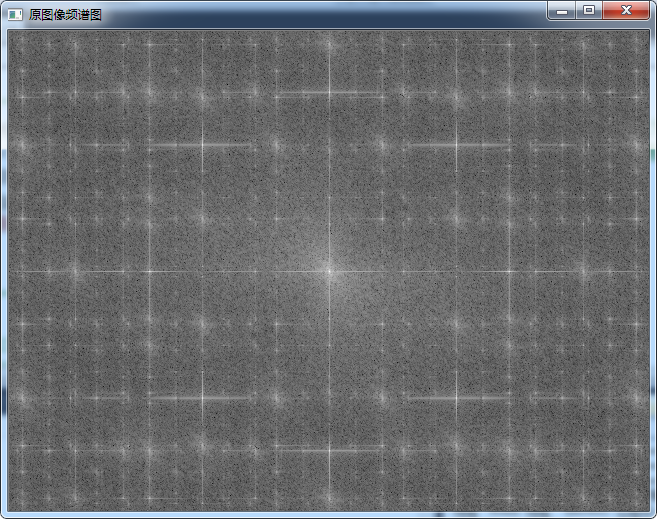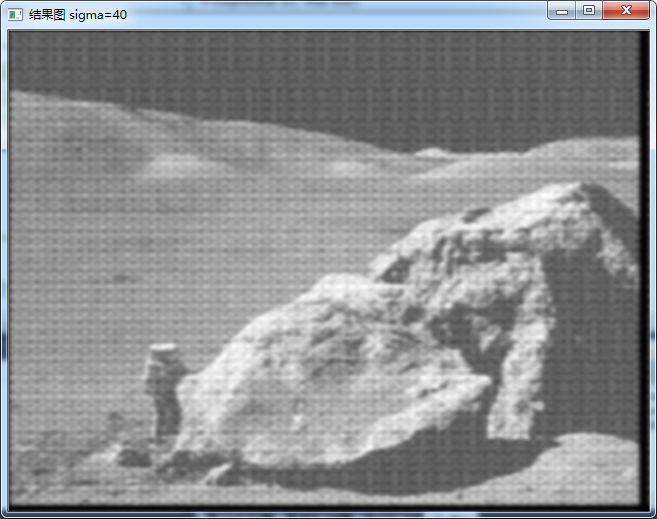注：1，结果的黑边为填充效果。      2，务必注意矩阵数据类型，做DFT，必须为浮点型，计算滤波器函数H（U，V）与DFT的乘积G（U，V）时，数据类型务必一致，包括通道数，单通道类型不能与多通道类型相乘，关于数据类型，可以参看这里。参考：https：//blog.csdn.net/dang_boy/article/details/76150067
展开全文• 基于频率域平滑滤波的数字图像处理方法研究论文，附源代码，能够实现图像的频率域平滑滤波效果，适用于图像去噪等。
• 图像增强处理：设计一套空间域与频率域结合的图像增强算法，处理以下任一组图片中的带噪声图像，去除噪声，提高图像质量。 （1）已知：噪声为随机噪声和周期噪声混合噪声； （2）要求： a）去噪处理后，计算均方误差...
• ## 图像去噪

千次阅读 2015-11-27 10:28:32
滤波，图像滤波可分为空间域滤波、频率域滤波两大类。 1空间域滤波降噪 空间域滤波降噪的基本原理是指直接对图像所在的二维空间进行处理，也就是直接 对每一像素点的灰度值进行处理，针对这一特点，空间域去噪...
• 一）空间域滤波与频率域滤波  1）空间域滤波  空间域滤波是指在图像空间中借助模板对图像领域进行操作，处理图像每一个像素值。主要分为线性滤波和非线性滤波两类，根据功能可分为平滑滤波器和锐化滤波器。...MATLAB
• 1. 空间滤波  空间滤波是指在图像空间中借助模板对图像领域进行操作，处理图像每一个像素值。主要分为线性滤波和非...二是去噪。锐化则可用高通滤波来实现，锐化的目的是为了增强被模糊的细节。  在matl
• ## 小波变换和小波阈值法去噪

万次阅读 多人点赞 2017-07-24 18:05:38
小波变换是一种信号的时间——尺度（时间——频率）分析方法，它具有多分辨分析的特点，而且在时频两都具有表征信号局部特征的能力，。在小波分析中经常用到近似和细节，近似表示信号的高尺度，即低频信息；细节...小波 连续小波变换 离散小波变换
• 该方法的滤波权重是根据距离中的像素相似度和图像像素的出现频率在固定窗口内以迭代方式计算的，但是没有空间的滤波权重。 其次，将所提出的方法与高斯滤波作为引擎相结合，以完成图像平滑的任务，因为在抑制...研究论文
• 提出一种基于小波内统计建模的低剂量计算机X 线断层(CT) 图像去噪新方法。利用非下采样Contourlet 变换(NSCT)获得具有平移不变性的多尺度、多方向频率子带；结合噪声特点,通过统计参数预置改进隐马尔可夫树(HMT)...
• 什么是傅里叶变换？ 推荐一个极其优秀的知乎文章，看完它，比说什么都强。...傅里叶变换在opencv中实现图像去噪 代码演示： #include "stdafx.h" #include <opencv2\opencv.hpp> #include <iostream&g
• 空间域滤波和频率域滤波 1.空间域滤波 空间域滤波是指在图像空间中借助模板对图像领域进行操作，处理图像每一个像素值。主要分为线性滤波和非线性滤波两类，根据功能可分为平滑滤波器和锐化滤波器。平滑可通过低通来...
• 1. 噪声产生的原因决定了它的分布特性。...一类是频率域去噪 3.中值滤波是一种最常用到的非线性平滑滤波方法，它的基本原理是将数字图像或序列中某点的值用该点邻域中各个点值得中值来替代。（利用一个活动的立体匹配 三维重建 滤波
• 图像增强处理：设计2套空间域与频率域结合的图像增强算法，处理以下两组图片中的带噪声图像，去除噪声，提高图像质量。 （1）已知：噪声为随机噪声和周期噪声混合噪声； （2）要求： a）去噪处理后，计算均方误差...
• 小波变换是一种信号的时间——尺度（时间——频率）分析方法，它具有多分辨分析的特点，而且在时频两都具有表征信号局部特征的能力，是一种窗口大小固定不变但其形状可改变，时间窗和频率窗都可以改变的时频局部化...matlab
• 假设图像退化过程被建模为一个退化函数和一个加性噪声项，对输入图像f(x,y)进行处理，产生退化后的图像g(x,y)。...等价的频率域表示为： 下图是图像退化/复原过程的模型： 噪声概率密度函数 考虑加性噪声，...图像降噪 自适应中值滤波器 高斯噪声 均值滤波器
• 频两均能表征信号局部特征的特点,采用小波分解和小波包分解对掘进机三方向振动信号进行分解重构,比较sym4小波,sym5小波和小波包对振动信号的去噪能力,选择sym4对振动信号进行处理,获取掘进机振动信号的特征频率和...
• 波变换是一种信号的时间——频率分析方法，它具有多分辨分析的特点，而且在时频两都具有表征信号局部特征的能力，是一种窗口大小固定不变但其形状可改变，时间窗和频率窗都可以改变的时频局部化分析方法。...
• 在分析多种时频分析方法的基础上，...研究结果表明，改进的极值均值模式分解法能够有效去除脑电信号的噪声部分，消除邻近频率的混叠影响和边界效应。对利用脑电信号诊断癫痫、缺血性脑损和睡眠监护有临床指导作用。
• 前言去年图像处理的DLL，有学弟问我做的思路，便放到博客里 github地址，欢迎star 图像增强处理：设计一套空间域与频率域结合的图像增强算法，处理以下任一组图片中的带噪声图像，去除噪声，提高图像质量。...
• 波动方程逆时偏移是目前比较精确的基于波动理论的深度偏移成像方法,但逆时偏移成像条件的运用会产生低频噪音,影响成像质量及层位的准确性。依据低频噪声的产生机理,运用交错网格有限差分法求取各向同性介质中的...